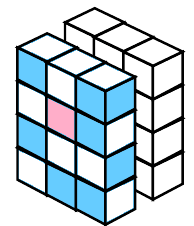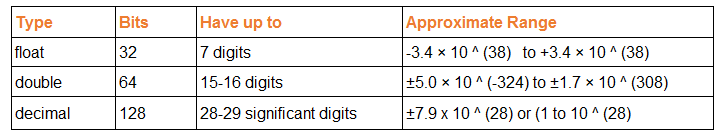# Decimal, Float and Double

## Decimal vs Double vs FloatThe Decimal, Double, and Float variable types are different in the way that they store the values. Precision is the main difference where float is a single precision (32 bit) floating point data type, double is a double precision (64 bit) floating point data type and decimal is a 128-bit floating point data type.

1. Float - 32 bit (7 digits)
2. Double - 64 bit (15-16 digits)
3. Decimal - 128 bit (28-29 significant digits)

## Difference between Decimal, Float and DoubleThe main difference is Floats and Doubles are binary floating point types and a Decimal will store the value as a floating decimal point type. So Decimals have much higher precision and are usually used within monetary (financial) applications that require a high degree of accuracy. But in performance wise Decimals are slower than double and float types.

Decimal can 100% accurately represent any number within the precision of the decimal format, whereas Float and Double, cannot accurately represent all numbers, even numbers that are within their respective formats precision.

## DecimalIn case of financial applications it is better to use Decimal types because it gives you a high level of accuracy and easy to avoid rounding errors

## Double

Double Types are probably the most normally used data type for real values, except handling money.

## Float

It is used mostly in graphic libraries because very high demands for processing powers, also used situations that can endure rounding errors.

### Approximate Range## Accuracy

1. Float is less accurate than Double and Decimal.
2. Double is more accurate than Float but less accurate than Decimal.
3. Decimal is more accurate than Float and Double.

NEXT.....String to DateTime